# NCERT Solutions for Class 10 Science Chapter 2

NCERT Solutions for Class 10 Science Chapter 2 – Acids, Bases and Salts, contains solutions to various questions in Exercises for Chapter 2. Acids, Bases and Salts Class 10 NCERT Solutions have been explained in a simple and easy to understand manner. We are providing all subjects which can be accessed by clicking here.

## Download NCERT Solutions for Class 10 Science Chapter 2 – Acids, Bases and Salts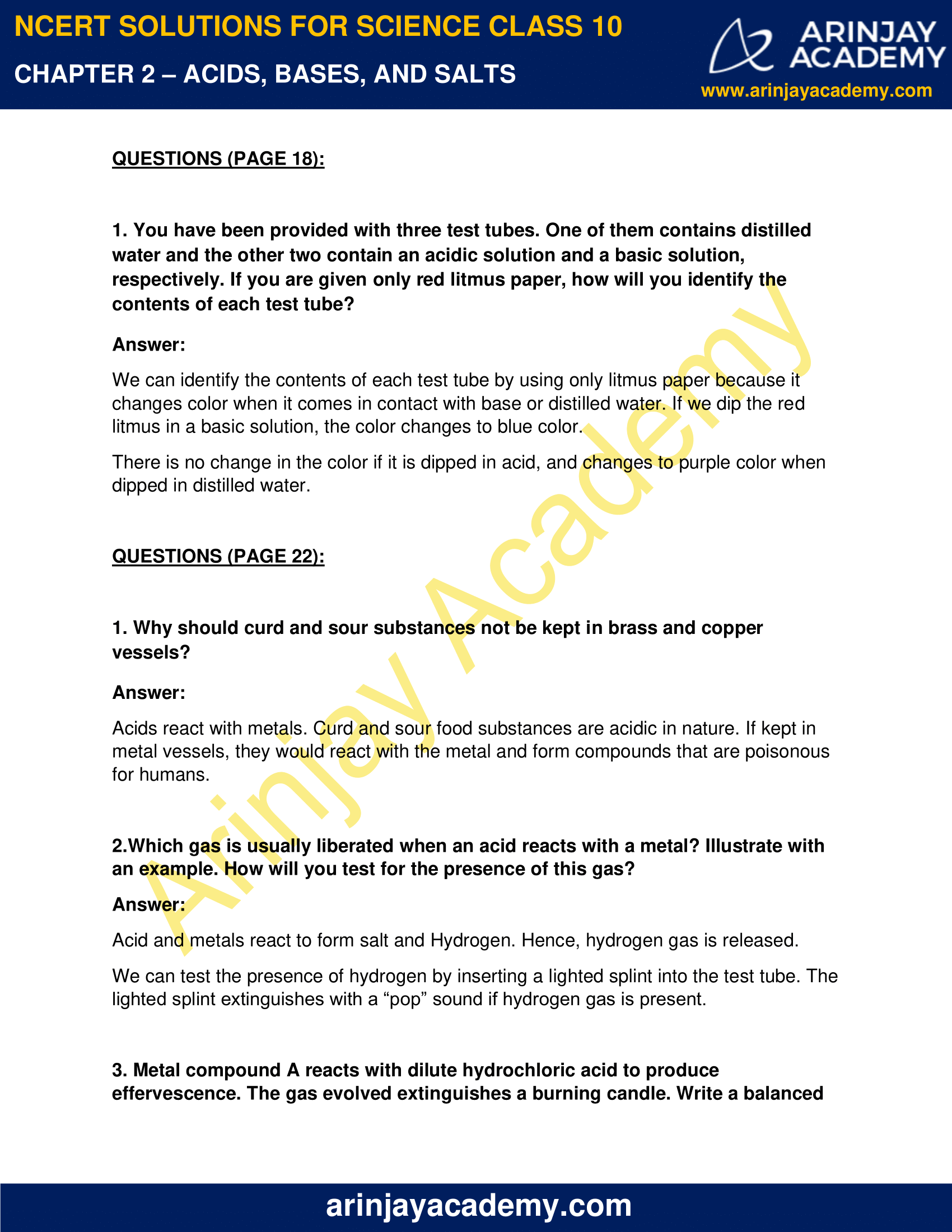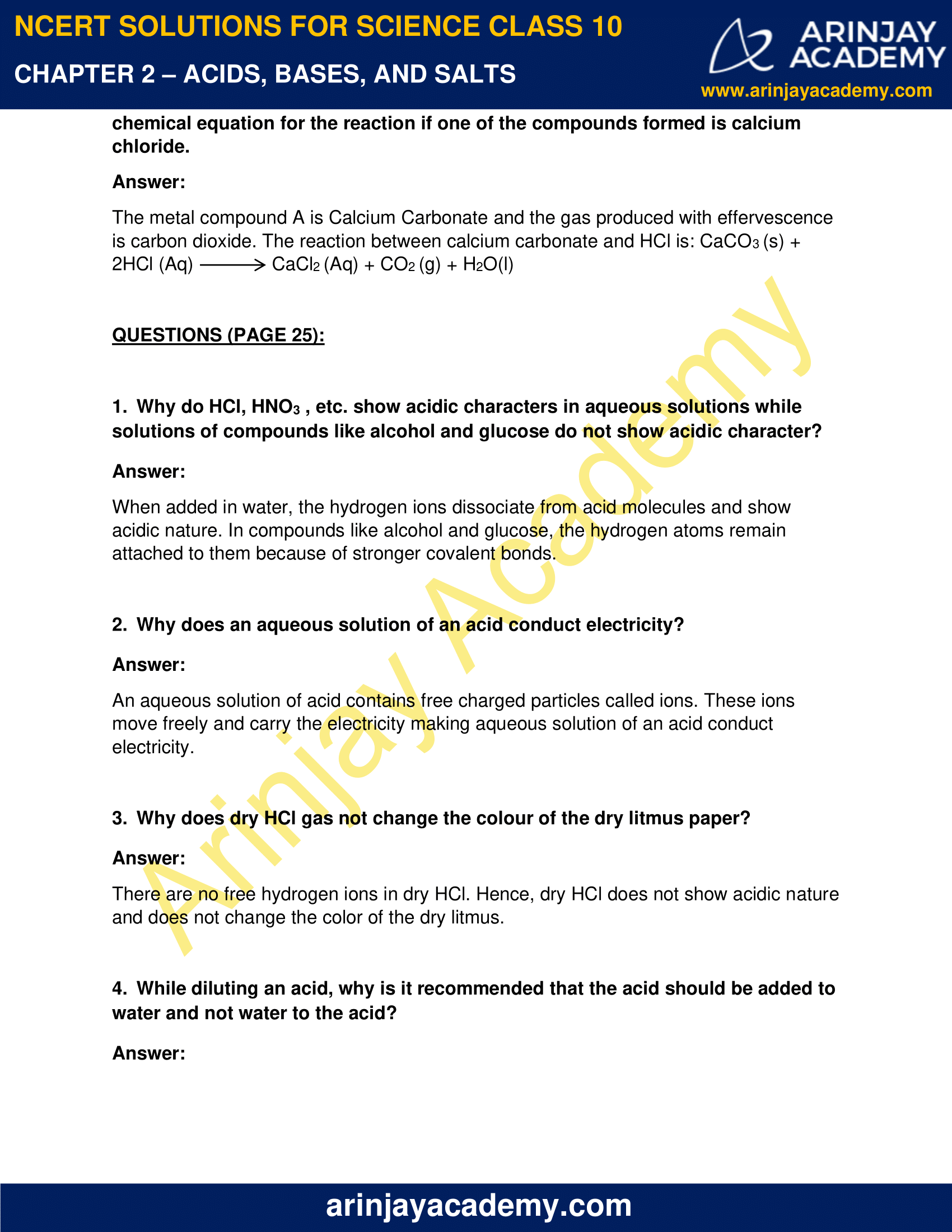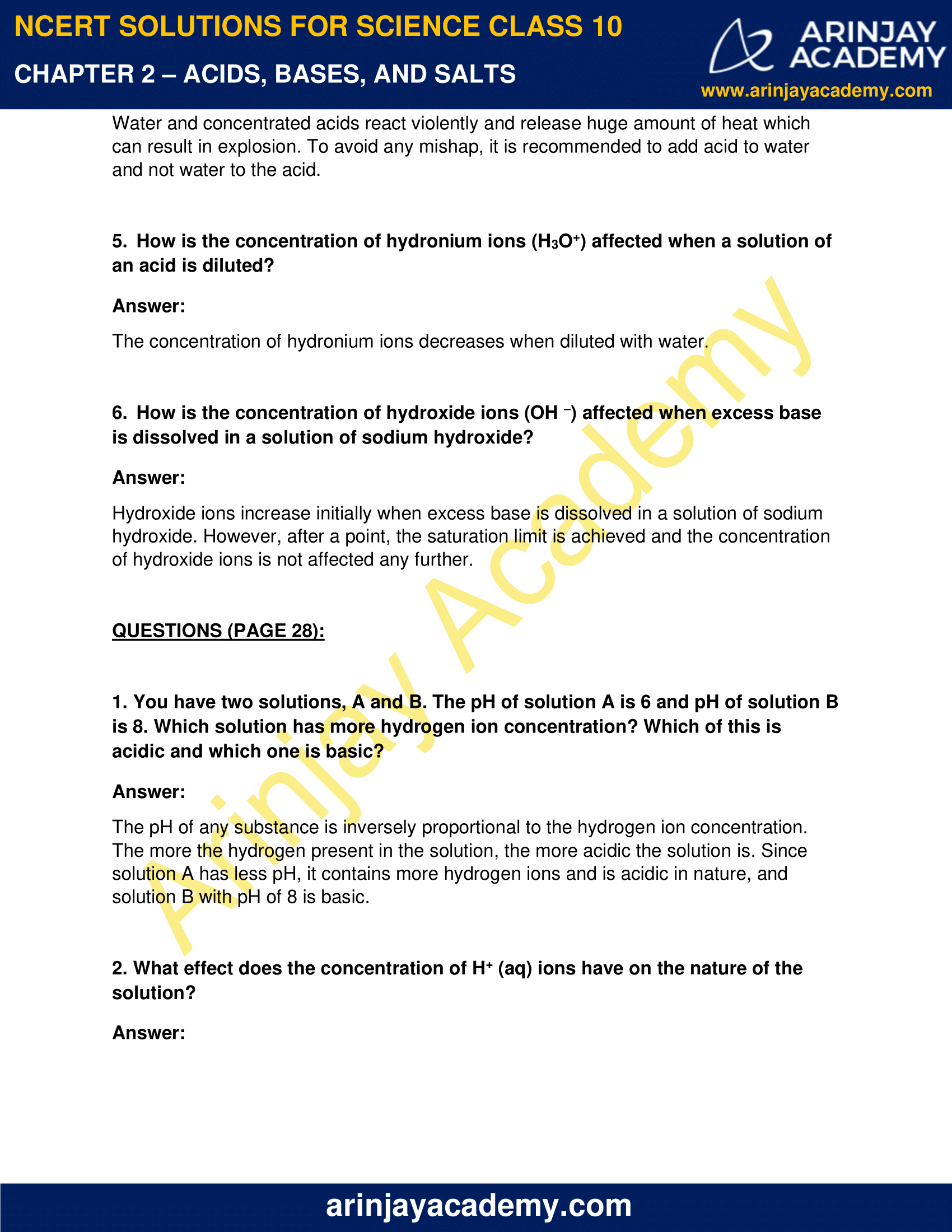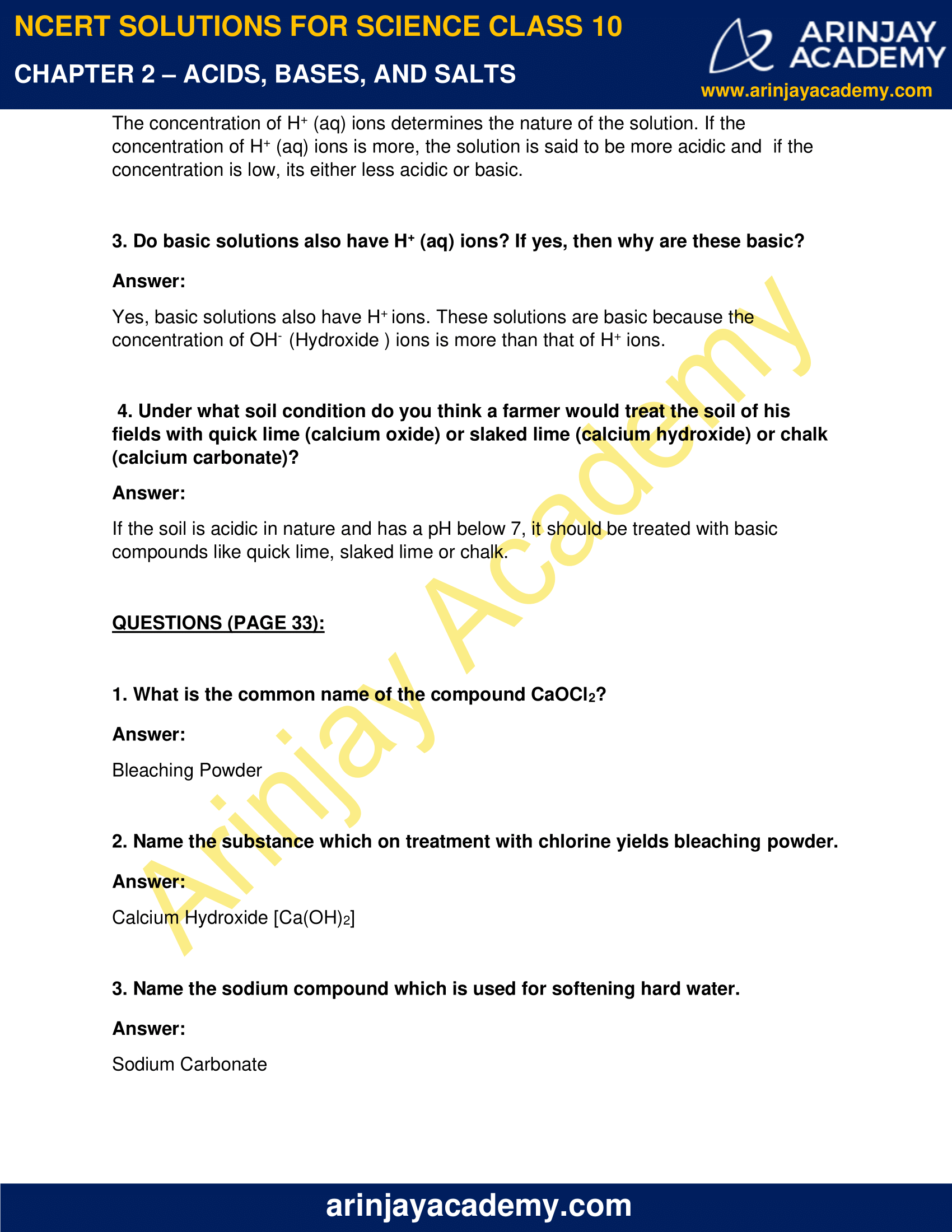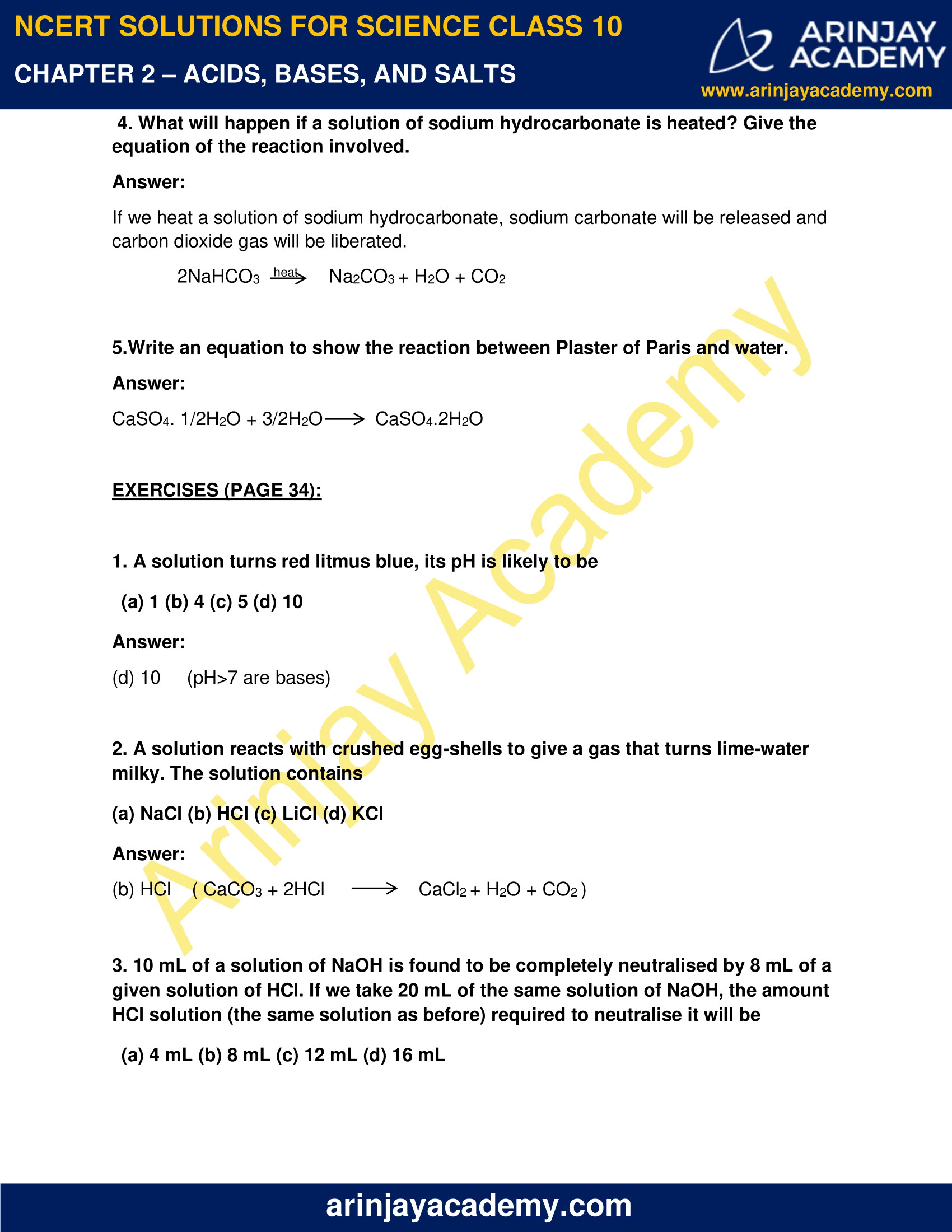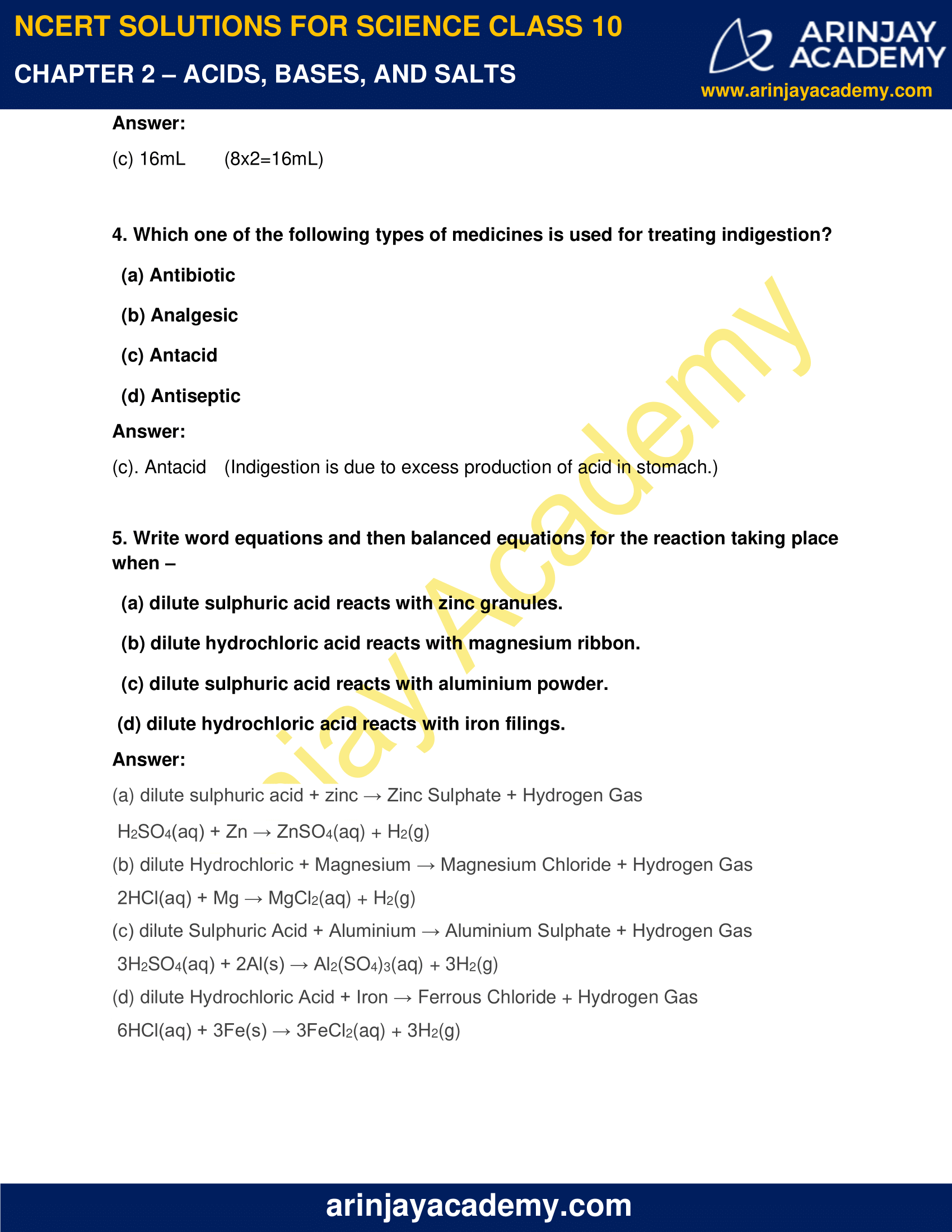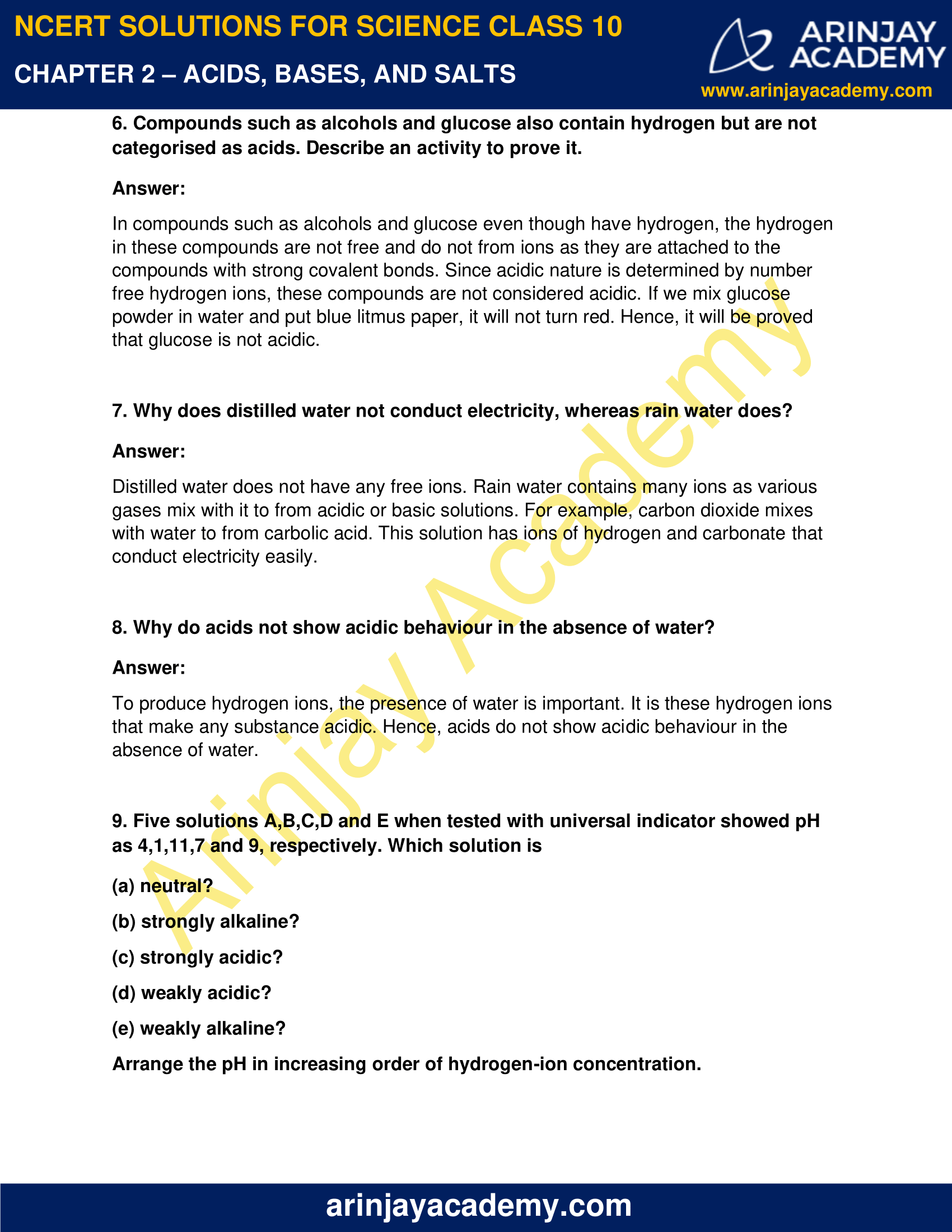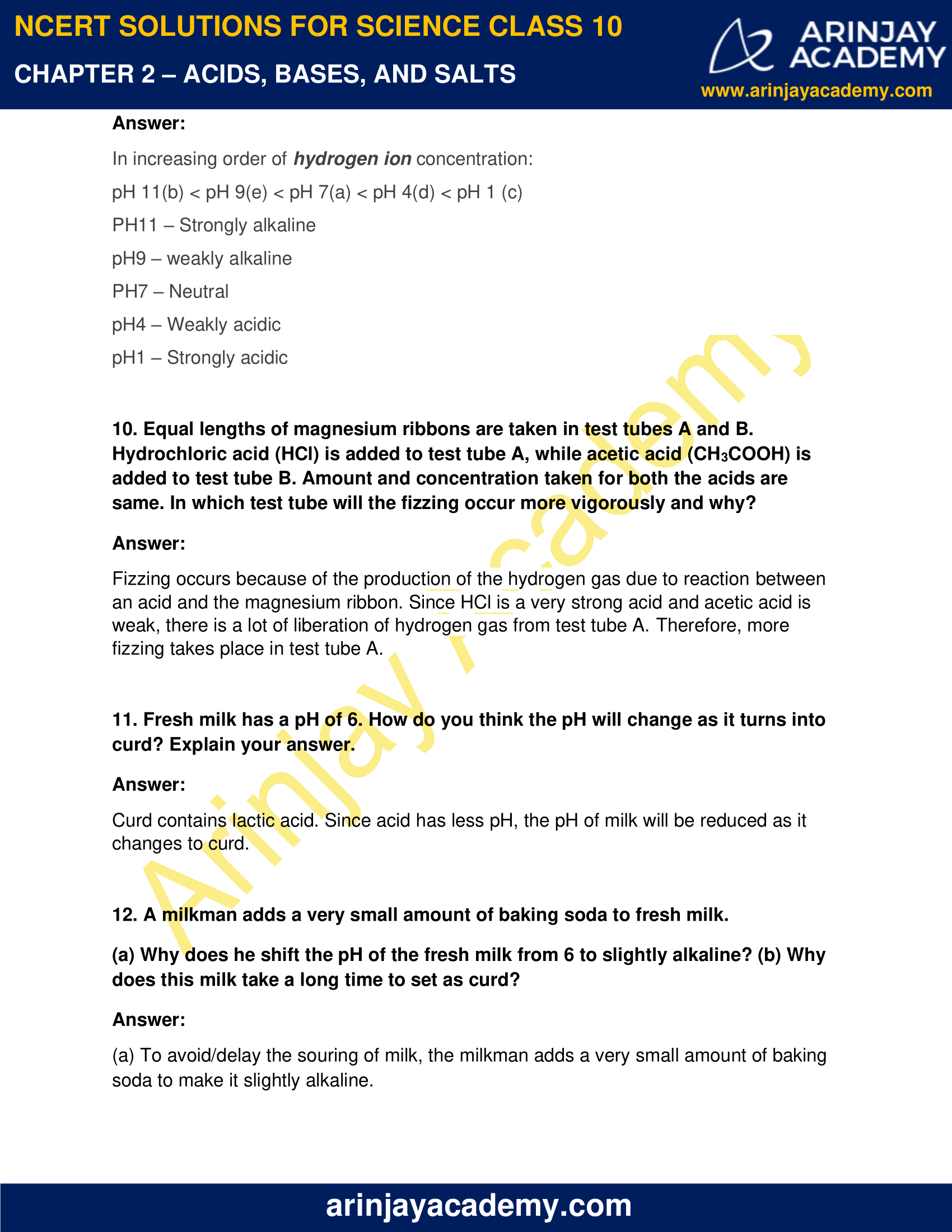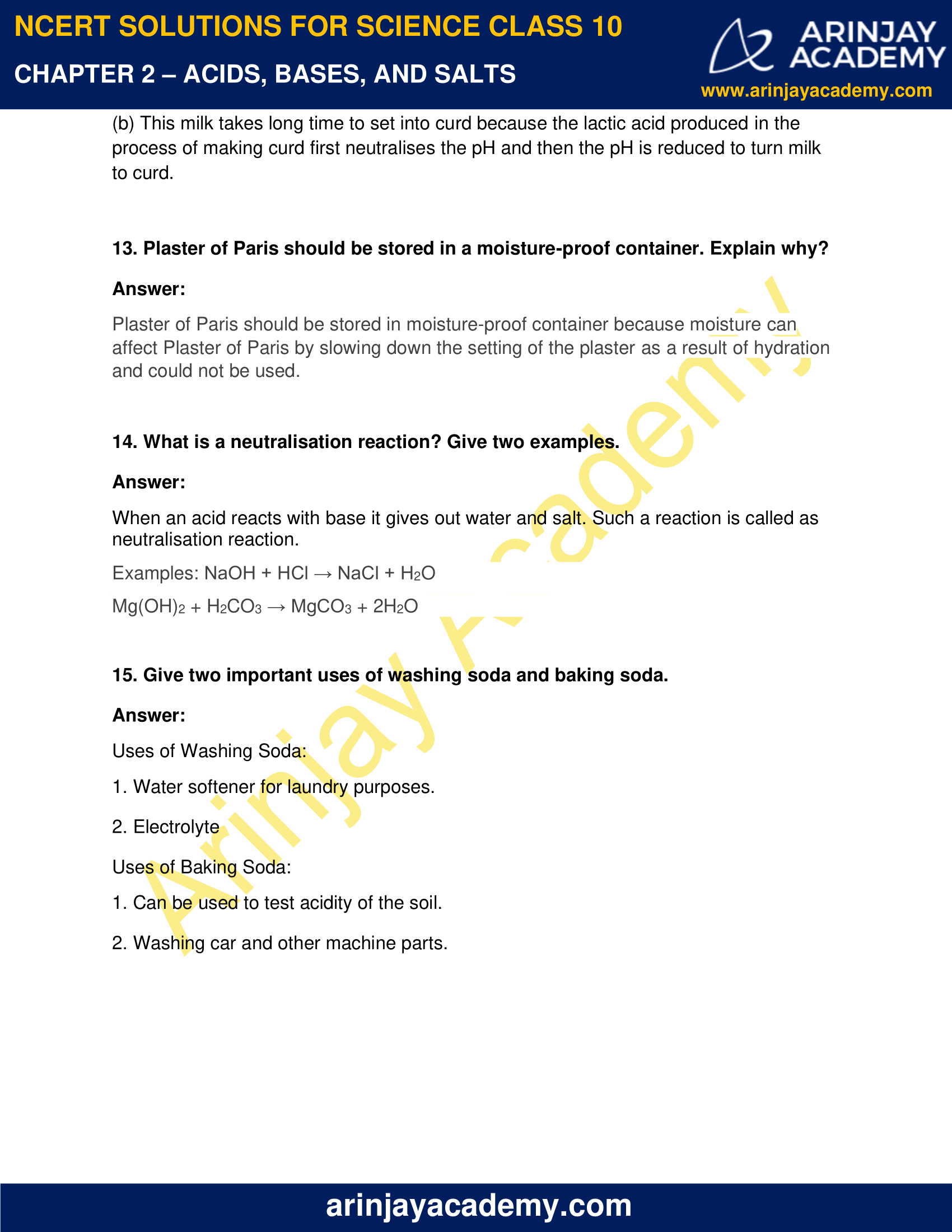### NCERT Solutions for Class 10 Science Chapter 2 – Acids, Bases and Salts – NCERT Exercises

NCERT Solutions for Class 10 Science Chapter 2 – Acids, Bases and Salts, Exercises includes all in text and exercise solved questions which helps you to understand the topic covered in Chapter 2 Acids, Bases and Salts Class 10, in a better manner to help you to score good marks in your examinations.

### NCERT Solutions for Class 10 Science Chapter 2 – Intext Questions – Page 18

1. You have been provided with three test tubes. One of them contains distilled water and the other two contain an acidic solution and a basic solution, respectively. If you are given only red litmus paper, how will you identify the contents of each test tube?

Answer:

We can identify the contents of each test tube by using only litmus paper because it changes color when it comes in contact with base or distilled water. If we dip the red litmus in a basic solution, the color changes to blue color.

There is no change in the color if it is dipped in acid, and changes to purple color when dipped in distilled water.

### Intext Questions – Page 22

1. Why should curd and sour substances not be kept in brass and copper vessels?

Answer:

Acids react with metals. Curd and sour food substances are acidic in nature. If kept in metal vessels, they would react with the metal and form compounds that are poisonous for humans.

2.Which gas is usually liberated when an acid reacts with a metal? Illustrate with an example. How will you test for the presence of this gas?

Answer:

Acid and metals react to form salt and Hydrogen. Hence, hydrogen gas is released.

We can test the presence of hydrogen by inserting a lighted splint into the test tube. The lighted splint extinguishes with a “pop” sound if hydrogen gas is present.

3. Metal compound A reacts with dilute hydrochloric acid to produce effervescence. The gas evolved extinguishes a burning candle. Write a balanced chemical equation for the reaction if one of the compounds formed is calcium chloride.

Answer:

The metal compound A is Calcium Carbonate and the gas produced with effervescence is carbon dioxide. The reaction between calcium carbonate and HCl is: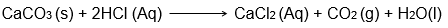### Intext Questions – Page 25

1. Why do HCl, HNO3 , etc. show acidic characters in aqueous solutions while solutions of compounds like alcohol and glucose do not show acidic character?

Answer:

When added in water, the hydrogen ions dissociate from acid molecules and show acidic nature. In compounds like alcohol and glucose, the hydrogen atoms remain attached to them because of stronger covalent bonds.

2. Why does an aqueous solution of an acid conduct electricity?

Answer:

An aqueous solution of acid contains free charged particles called ions. These ions move freely and carry the electricity making aqueous solution of an acid conduct electricity.

3. Why does dry HCl gas not change the colour of the dry litmus paper?

Answer:

There are no free hydrogen ions in dry HCl. Hence, dry HCl does not show acidic nature and does not change the color of the dry litmus.

4. While diluting an acid, why is it recommended that the acid should be added to water and not water to the acid?

Answer:

Water and concentrated acids react violently and release huge amount of heat which can result in explosion. To avoid any mishap, it is recommended to add acid to water and not water to the acid.

5. How is the concentration of hydronium ions (H3O+) affected when a solution of an acid is diluted?

Answer:

The concentration of hydronium ions decreases when diluted with water.

6. How is the concentration of hydroxide ions (OH ) affected when excess base is dissolved in a solution of sodium hydroxide?

Answer:

Hydroxide ions increase initially when excess base is dissolved in a solution of sodium hydroxide. However, after a point, the saturation limit is achieved and the concentration of hydroxide ions is not affected any further.

### Intext Questions – Page 28

1. You have two solutions, A and B. The pH of solution A is 6 and pH of solution B is 8. Which solution has more hydrogen ion concentration? Which of this is acidic and which one is basic?

Answer:

The pH of any substance is inversely proportional to the hydrogen ion concentration. The more the hydrogen present in the solution, the more acidic the solution is. Since solution A has less pH, it contains more hydrogen ions and is acidic in nature, and solution B with pH of 8 is basic.

2. What effect does the concentration of H+ (aq) ions have on the nature of the solution?

Answer:

The concentration of H+ (aq) ions determines the nature of the solution. If the concentration of H+ (aq) ions is more, the solution is said to be more acidic and  if the concentration is low, its either less acidic or basic.

3. Do basic solutions also have H+ (aq) ions? If yes, then why are these basic?

Answer:

Yes, basic solutions also have H+ ions. These solutions are basic because the concentration of OH–  (Hydroxide ) ions is more than that of H+ ions.

4. Under what soil condition do you think a farmer would treat the soil of his fields with quick lime (calcium oxide) or slaked lime (calcium hydroxide) or chalk (calcium carbonate)?

Answer:

If the soil is acidic in nature and has a pH below 7, it should be treated with basic compounds like quick lime, slaked lime or chalk.

### NCERT Solutions for Class 10 Science Chapter 2 – Intext Questions – Page 33

1. What is the common name of the compound CaOCl2?

Answer:

Bleaching Powder

2. Name the substance which on treatment with chlorine yields bleaching powder.

Answer:

Calcium Hydroxide [Ca(OH)2]

3. Name the sodium compound which is used for softening hard water.

Answer:

Sodium Carbonate

4. What will happen if a solution of sodium hydrocarbonate is heated? Give the equation of the reaction involved.

Answer:

If we heat a solution of sodium hydrocarbonate, sodium carbonate will be released and carbon dioxide gas will be liberated.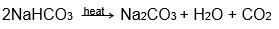5.Write an equation to show the reaction between Plaster of Paris and water.

Answer: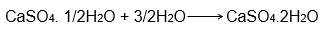### NCERT Solutions for Class 10 Science Chapter 2 – NCERT Exercise – Page 34

1. A solution turns red litmus blue, its pH is likely to be

(a) 1
(b) 4
(c) 5
(d) 10

Answer:

(d) 10 (pH>7 are bases)

2. A solution reacts with crushed egg-shells to give a gas that turns lime-water milky. The solution contains

(a) NaCl
(b) HCl
(c) LiCl
(d) KCl

Answer:

(b) HCl ( CaCO3 + 2HCl ⇒ CaCl2 + H2O + CO2 )

3. 10 mL of a solution of NaOH is found to be completely neutralised by 8 mL of a given solution of HCl. If we take 20 mL of the same solution of NaOH, the amount HCl solution (the same solution as before) required to neutralise it will be

(a) 4 mL
(b) 8 mL
(c) 12 mL
(d) 16 mL

Answer:

(c) 16mL (8×2=16mL)

4. Which one of the following types of medicines is used for treating indigestion?

(a) Antibiotic
(b) Analgesic
(c) Antacid
(d) Antiseptic

Answer:

(c). Antacid    (Indigestion is due to excess production of acid in stomach.)

5. Write word equations and then balanced equations for the reaction taking place when –

(a) dilute sulphuric acid reacts with zinc granules.

(b) dilute hydrochloric acid reacts with magnesium ribbon.

(c) dilute sulphuric acid reacts with aluminium powder.

(d) dilute hydrochloric acid reacts with iron filings.

Answer:

(a) dilute sulphuric acid + zinc → Zinc Sulphate + Hydrogen Gas

H2SO4(aq) + Zn → ZnSO4(aq) + H2(g)

(b) dilute Hydrochloric + Magnesium → Magnesium Chloride + Hydrogen Gas

2HCl(aq) + Mg → MgCl2(aq) + H2(g)

(c) dilute Sulphuric Acid + Aluminium → Aluminium Sulphate + Hydrogen Gas

3H2SO4(aq) + 2Al(s) → Al2(SO4)3(aq) + 3H2(g)

(d) dilute Hydrochloric Acid + Iron → Ferrous Chloride + Hydrogen Gas

6HCl(aq) + 3Fe(s) → 3FeCl2(aq) + 3H2(g)

6. Compounds such as alcohols and glucose also contain hydrogen but are not categorised as acids. Describe an activity to prove it.

Answer:

In compounds such as alcohols and glucose even though have hydrogen, the hydrogen in these compounds are not free and do not from ions as they are attached to the compounds with strong covalent bonds. Since acidic nature is determined by number free hydrogen ions, these compounds are not considered acidic. If we mix glucose powder in water and put blue litmus paper, it will not turn red. Hence, it will be proved that glucose is not acidic.

7. Why does distilled water not conduct electricity, whereas rain water does?

Answer:

Distilled water does not have any free ions. Rain water contains many ions as various gases mix with it to from acidic or basic solutions. For example, carbon dioxide mixes with water to from carbolic acid. This solution has ions of hydrogen and carbonate that conduct electricity easily.

8. Why do acids not show acidic behaviour in the absence of water?

Answer:

To produce hydrogen ions, the presence of water is important. It is these hydrogen ions that make any substance acidic. Hence, acids do not show acidic behaviour in the absence of water.

9. Five solutions A,B,C,D and E when tested with universal indicator showed pH as 4,1,11,7 and 9, respectively. Which solution is

(a) neutral?

(b) strongly alkaline?

(c) strongly acidic?

(d) weakly acidic?

(e) weakly alkaline?

Arrange the pH in increasing order of hydrogen-ion concentration.

Answer:

In increasing order of hydrogen ion concentration:

pH 11(b) < pH 9(e) < pH 7(a) < pH 4(d) < pH 1 (c)

PH11 – Strongly alkaline

pH9 – weakly alkaline

PH7 – Neutral

pH4 – Weakly acidic

pH1 – Strongly acidic

10. Equal lengths of magnesium ribbons are taken in test tubes A and B. Hydrochloric acid (HCl) is added to test tube A, while acetic acid (CH3COOH) is added to test tube B. Amount and concentration taken for both the acids are same. In which test tube will the fizzing occur more vigorously and why?

Answer:

Fizzing occurs because of the production of the hydrogen gas due to reaction between an acid and the magnesium ribbon. Since HCl is a very strong acid and acetic acid is weak, there is a lot of liberation of hydrogen gas from test tube A. Therefore, more fizzing takes place in test tube A.

11. Fresh milk has a pH of 6. How do you think the pH will change as it turns into curd? Explain your answer.

Answer:

Curd contains lactic acid. Since acid has less pH, the pH of milk will be reduced as it changes to curd.

12. A milkman adds a very small amount of baking soda to fresh milk.

(a) Why does he shift the pH of the fresh milk from 6 to slightly alkaline?
(b) Why does this milk take a long time to set as curd?

Answer:

(a) To avoid/delay the souring of milk, the milkman adds a very small amount of baking soda to make it slightly alkaline.

(b) This milk takes long time to set into curd because the lactic acid produced in the process of making curd first neutralises the pH and then the pH is reduced to turn milk to curd.

13. Plaster of Paris should be stored in a moisture-proof container. Explain why?

Answer:

Plaster of Paris should be stored in moisture-proof container because moisture can affect Plaster of Paris by slowing down the setting of the plaster as a result of hydration and could not be used.

14. What is a neutralisation reaction? Give two examples.

Answer:

When an acid reacts with base it gives out water and salt. Such a reaction is called as neutralisation reaction.

Examples: NaOH + HCl → NaCl + H2O

Mg(OH)2 + H2CO3 → MgCO3 + 2H2O

15. Give two important uses of washing soda and baking soda.

Answer:

Uses of Washing Soda:

1. Water softener for laundry purposes.
2. Electrolyte

Uses of Baking Soda:

1. Can be used to test acidity of the soil.
2. Washing car and other machine parts.

### Topics Covered in Acids, Bases and Salts Class 10 Science

• Chemical Properties of Acids and Bases
• Acids and Bases in the Laboratory
• How do Acids and Bases React with Metals?
• How do Metal Carbonates and Metal Hydrogencarbonates React with Acids?
• How do Acids and Bases React with each other?
• Reaction of Metallic Oxides with Acids
• Reaction of a Non-metallic Oxide with Base
• Common Properties in Acids and bases
• Acid or Base Solutions
• Salts